Electricity & Megnetism

# Electricity

Electricity is the name given to phenomena that occur to electrical charges and electrically charged bodies. The term electricity is also used to refer to a large area of ​​knowledge in physics, but it also applies to electrical energy — a form of energy that makes possible the operation of countless electrical devices.

In nature, there is an enormous amount of physical, chemical and biological phenomena that are explained by the interaction between charges, therefore, a good understanding of electricity makes it possible to understand many fundamental processes, often required by the current models of tests such as the National Education Exam. Medium (Enem).

Electricity originates from electrical charges , which are properties of subatomic particles such as protons and electrons. These two particles have the same amount of electrical charge, about 1.6 x 10 -19 C, however, they have opposite signs.

Since it is not possible for there to be fractions of protons or electrons inside atoms, we say that electric charge is a discrete or quantized property , since all the electric charge present in an electrified body is equal to an integer multiple of the electric charge. fundamental, as shown in the following expression: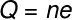Q – electrical charge

n – number of missing or excess electrons

## Electricity and Coulomb’s Law

According to Coulomb’s law , electric charges of opposite signs tend to attract each other, thanks to the action of electric force . This force, in turn, depends on a property of charges known as the electric field .

Like the electric force, the electric field is a vector property , that is, at each point in space around an electric charge there is a magnitude, a direction and an electric field direction. Furthermore, according to the SI , the unit of measurement of electric field is the newton per coulomb (N/C).

Coulomb’s law is expressed in the following image. According to this law, the force of attraction or repulsion between charges is equal to the product of these charges multiplied by an electrostatic constant (k 0 ) and divided by the square of the distance separating them: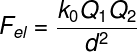0 – electrostatic vacuum constant (9.10 9 Nm²/C²)

1 and Q 2 – interacting electrical charges (C)

d – distance between electrical charges (m)

## Electricity and electric field

When a free electric charge is abandoned in a region where there is an electric field, it moves, giving rise to electric current , which can be understood as the movement of electric charges due to a potential difference . In the following figure, you can check the formula used to calculate the electric current.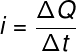i – electric current (A)

ΔQ – electrical charge (C)

Δt – time interval (s)

The electric field is a property of the charge that depends on the magnitude of that charge and that is inversely proportional to the square of the distance between the charge and the point at which the electric field strength is to be measured. The electric field formula is shown below, note :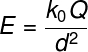E – electric field (N/C)

## Electricity and electrical potential

We call electric potential the amount of energy, in joules , that each charge obtains when disposed at some point in an electric field. This property does not depend on the charge itself, but on the strength of the electric field .

We commonly call the electrical potential electrical voltage or potential difference, and some people use the colloquial term voltage. Electric potential is a scalar quantity, whose unit of measurement, according to the SI, is the volt (V), which is equivalent to joules per coulomb (J/C).

The formula for electric potential is this, check it out: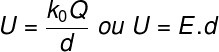U – electric potential (V)

## electricity generation

Most of the electricity used around the world is generated through the phenomenon of electromagnetic induction , mathematically described by the Faraday-Lenz law . According to such a law, when the “number” of magnetic field lines passing through a closed conductor varies with time, an electric current arises in the conductor , so as to compensate for the variation in the number of magnetic field lines .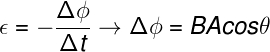ε – induced electromotive force or induced voltage (V)

ΔΦ – variation of magnetic field flux (Wb – weber)

B – magnetic field strength (T – tesla)

A – area (m²)

The electricity generated in hydroelectric , thermoelectric , nuclear , wind and geothermal plants is produced in the same way: mechanical energy ( kinetic and potential energy) is transformed into electrical energy, through the rotation of a large dynamo.

The basic structure of a dynamo consists of a coil of conducting wires capable of turning, arranged inside a large magnet. The variation of the angle at which the magnetic field lines penetrate the coil causes an alternating electric current to appear in it.

## electricity consumption

Electricity consumption consists of the amount of energy consumed by the use of electronic devices . In Brazil, electricity consumption is measured in the kilowatt-hour unit (1 kWh = 1000 Wh), which is equivalent to an amount of energy equal to 3.6 x 10 6 J .

In order to calculate the electrical energy consumed by some device, we need to know two things — its power , in kW (if the power is defined in watts, we must divide it by 1000), and the time that that device operates, in hours, as shown in the following formula: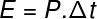E – electrical energy consumed (kWh)

P – electrical power (kW)

Δt – time interval (h)

Finally, to calculate the electricity consumption, the monthly period is generally taken into account. Therefore, it is important to remember that the device’s daily operating time is usually multiplied by 30, in order to find out how much energy was consumed over the course of a month , so that, then, the energy consumed is multiplied , in kWh, at the price of each kWh.

## Exercises solved on electricity

Question 1) (Unicamp) (Adapted) An example of technological development, with a view to fast recharging, is the prototype of a lithium-ion battery, with a three-dimensional structure. Assume that a battery, initially discharged, is charged with an average current of 3.2 A until it reaches its maximum charge of Q = 0.8 Ah. The time taken to charge the battery is:

a) 240 minutes

b) 90 minutes

c) 15 minutes

d) 4 minutes

e) 5 minutes

feedback :

Resolution: letter C

Let’s use the electric current formula to calculate the time in hours required for the battery to be fully charged, check: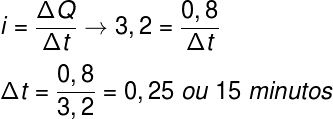According to the calculation, the time required for the battery to be fully charged is 0.25 hours or 15 minutes, which corresponds to a quarter of an hour. Therefore, the correct alternative is letter C.

Question 2) (Puccamp) A few years ago, residential lighting was predominantly done using incandescent lamps. Currently, paying attention to the policy of preserving natural resources, these lamps are being replaced by much more economical ones, such as compact fluorescent and LED ones. In a residence, 10 incandescent lamps of 100 W were used, which were on, on average, 5 hours a day. These lamps were replaced by 10 compact fluorescent lamps that consume 20 W each and are also on, on average, 5 hours a day.

Adopting the value of R\$ 0.40 for the kilowatt-hour price, the savings that this exchange provides, in a 30-day month, is:

a) BRL 18

b) BRL 48

c) BRL 60

d) BRL 120

e) BRL 248

Template : Letter B

Resolution:

We are going to use the electricity consumption formula to solve the question, for that, we will do the calculation for the two cases, in which incandescent and fluorescent lamps are used. Then, to obtain the energy savings, we must calculate the difference in energy consumption and then multiply it by the kWh value, in reais, check: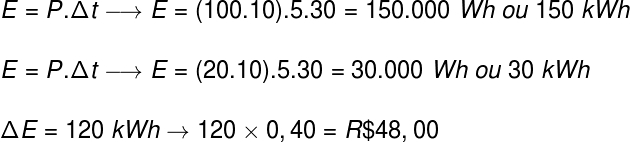Based on the calculation performed, we found that the savings in electricity, at the end of 30 days, was R\$ 48, therefore, the correct alternative is the letter B.